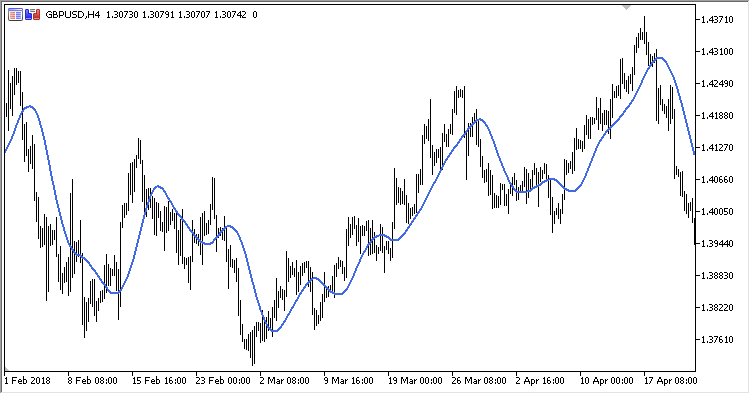Interesting script?
So post a link to it -
let others appraise it

You liked the script? Try it in the MetaTrader 5 terminal# SineWMA - indicator for MetaTrader 5

Views:
1230
Rating:
Published:
2018.12.18 19:15

Sine Weighted Moving Average indicator is a sine-weighted moving average.

It has two configurable parameters:

• Period - calculation period
• Applied price

Calculation:

SineWMA = Sum / Weight

where:

Sum= Price[i-N+1] * Sin(PI*(N)/(N+1)) + Price[i-N+2] * Sin(PI*(N-1)/(N+1)) + … + Price[i] * Sin(PI*1/(N+1))
Weight = Sin(PI*(N)/(N+1)) + Sin(PI*(N-1)/(N+1)) + … + Sin(PI*1/(N+1))Translated from Russian by MetaQuotes Software Corp.
Original code: https://www.mql5.com/ru/code/22509Smoothed_RSI

Smoothed RSI indicatorSRSI

Slow RSI indicatorNormalized_Moving_Average_Slope

Normalized Moving Average Slope indicatorMACD_Flat_Trend

MACD Flat Trend indicator